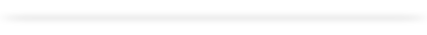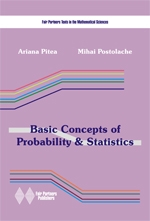Editura
Colectii
Autori
Contact0 produse in cos# Basic Concepts of Probability & StatisticsAutori: Pitea Ariana, Postolache Mihai Colectia: Fair Partners Texts in the Mathematical Sciences ISBN: 978-973-1877-79-2 Pagini: 198+viii An aparitie: 2012 Descriere scurta: Second revised edition Many important practical phenomena may be modeled by means of probabilistic argu-ments or using the methods of mathematical statistics. For instance, such models can be found in engineering, queuing theory, games theory, information theory, medicine etc. In order to under-stand these phenomena necessarily implies being able to know the theoretical background and to solve some applied problems that models them. The object of our book is to introduce the reader in the wonderful world of the theory of probability and statistics using basic theory and applied problems. The book can be used both as a study aid in probability theory and statistics  and as a collection of problems (contains over 400 worked exercises and problems). Problems and exercises are systematically selected and arranged in compliance with the major sections of the course in technical universities. Some exercises of a theoretical nature are included. Particular attention is given to questions which are not sufficiently treated in the available textbooks and which, as is shown by our experience, are poorly understood by students (for instance the geometric probabilities, DeMoivre-Laplace Theorem, the links with the reliability theory, the positive definite functions and so on). The theoretical details at the beginning of each chapter make the text sufficiently self contained to enable independent research. Also, the drawings that accompany the text serve to help the reader in analyzing the theoretical material and problems.